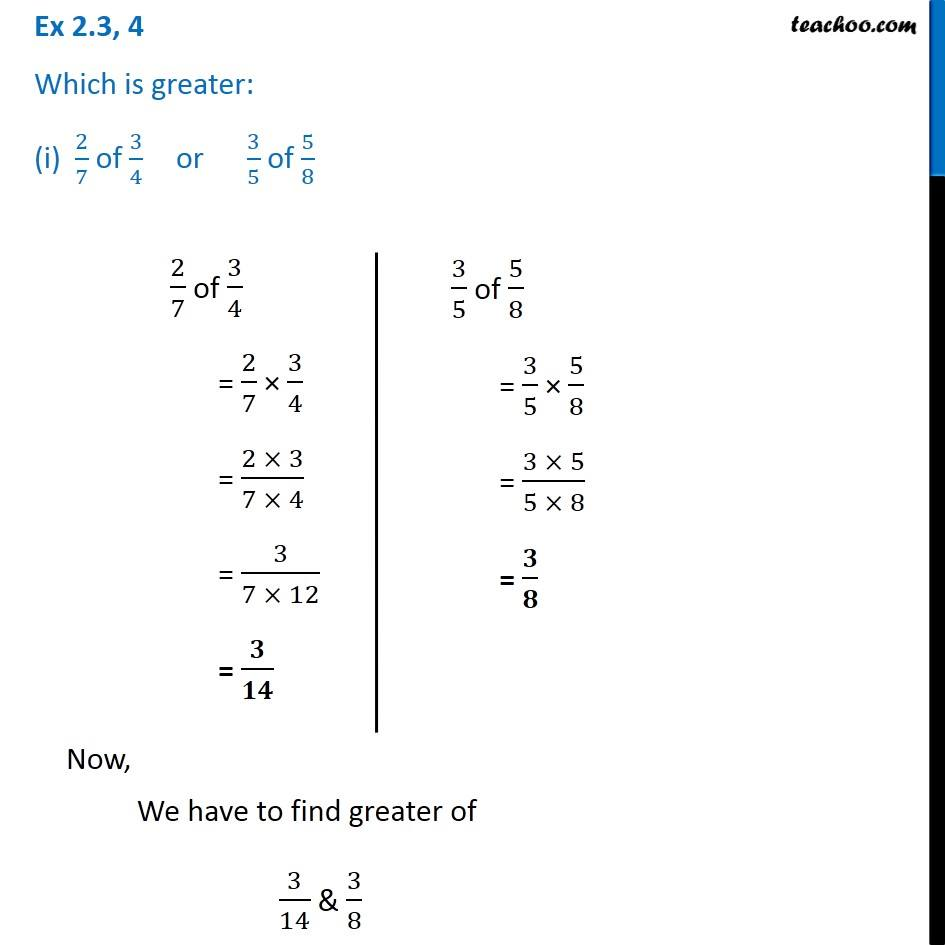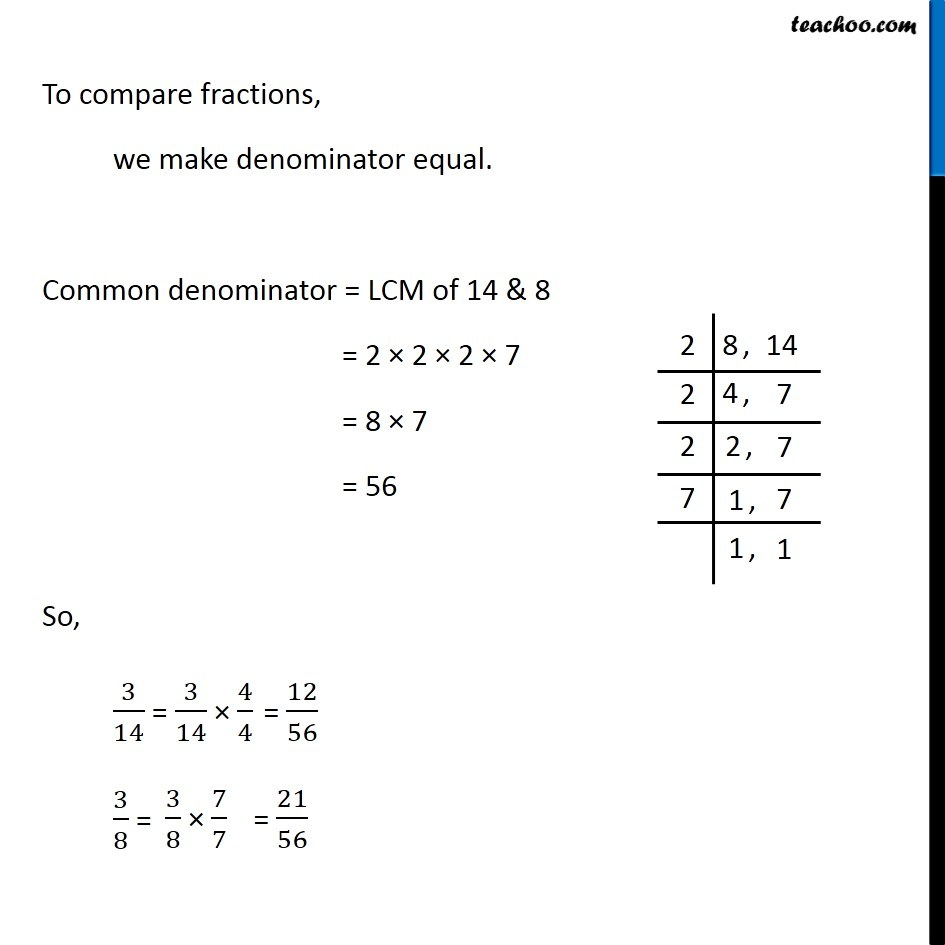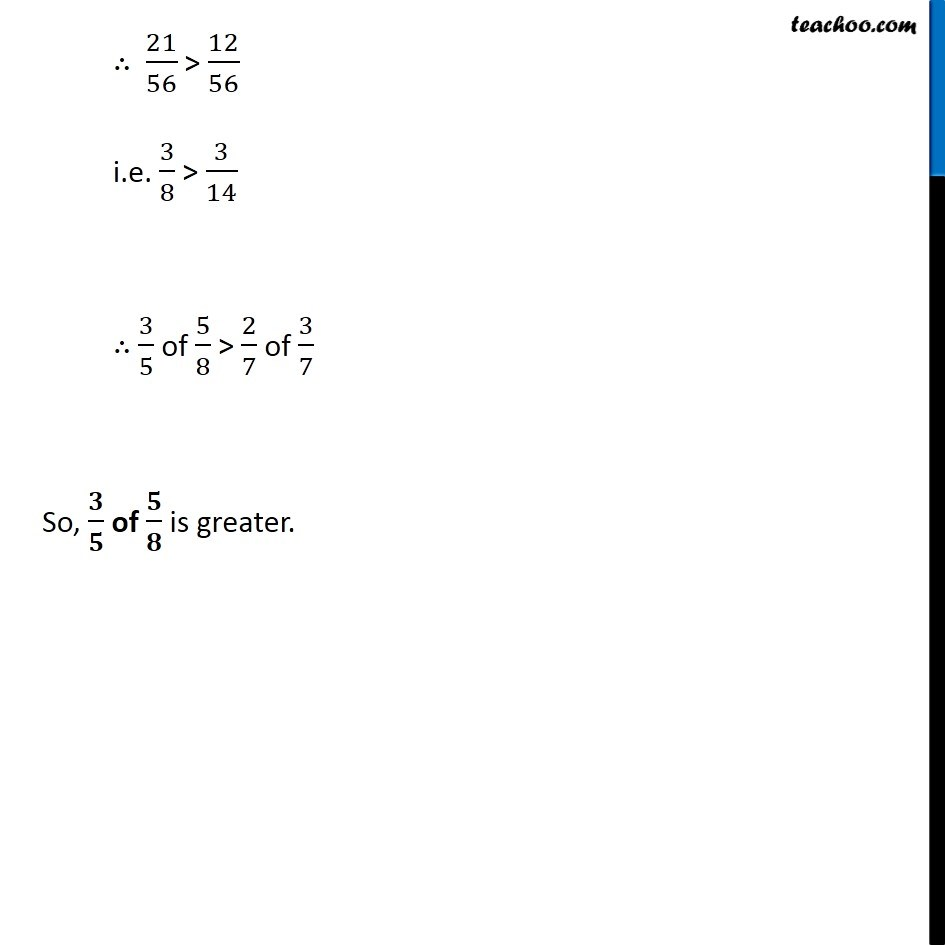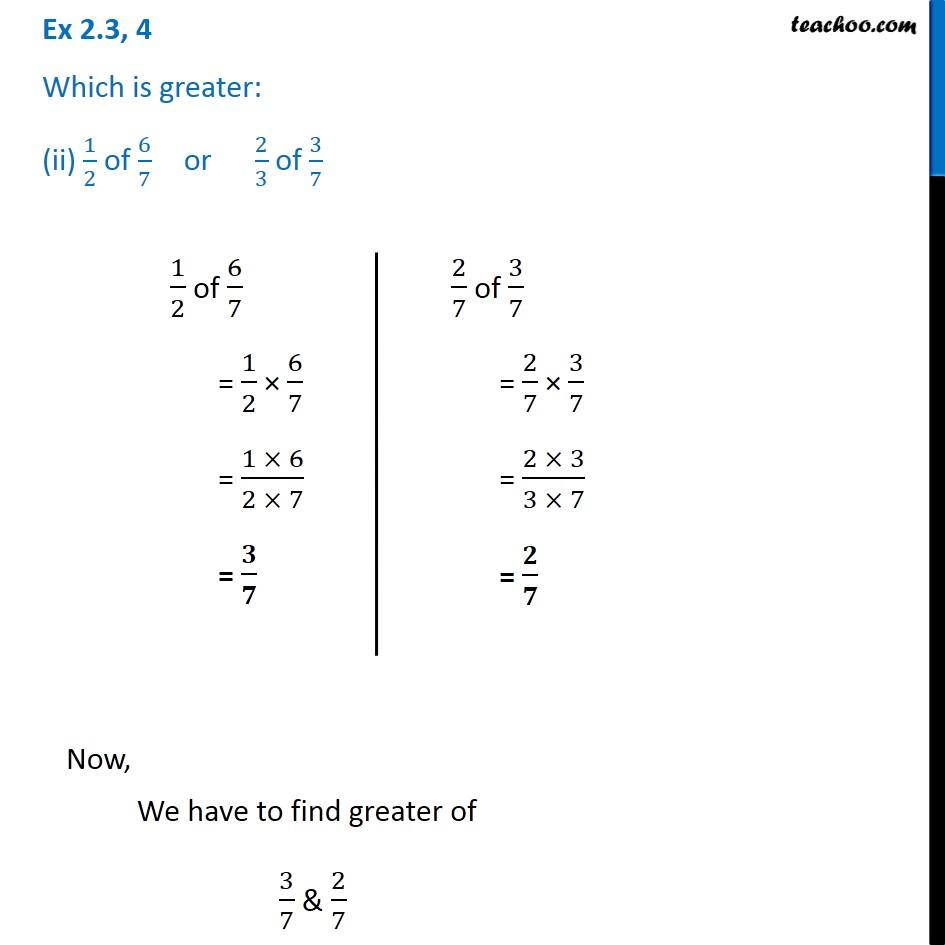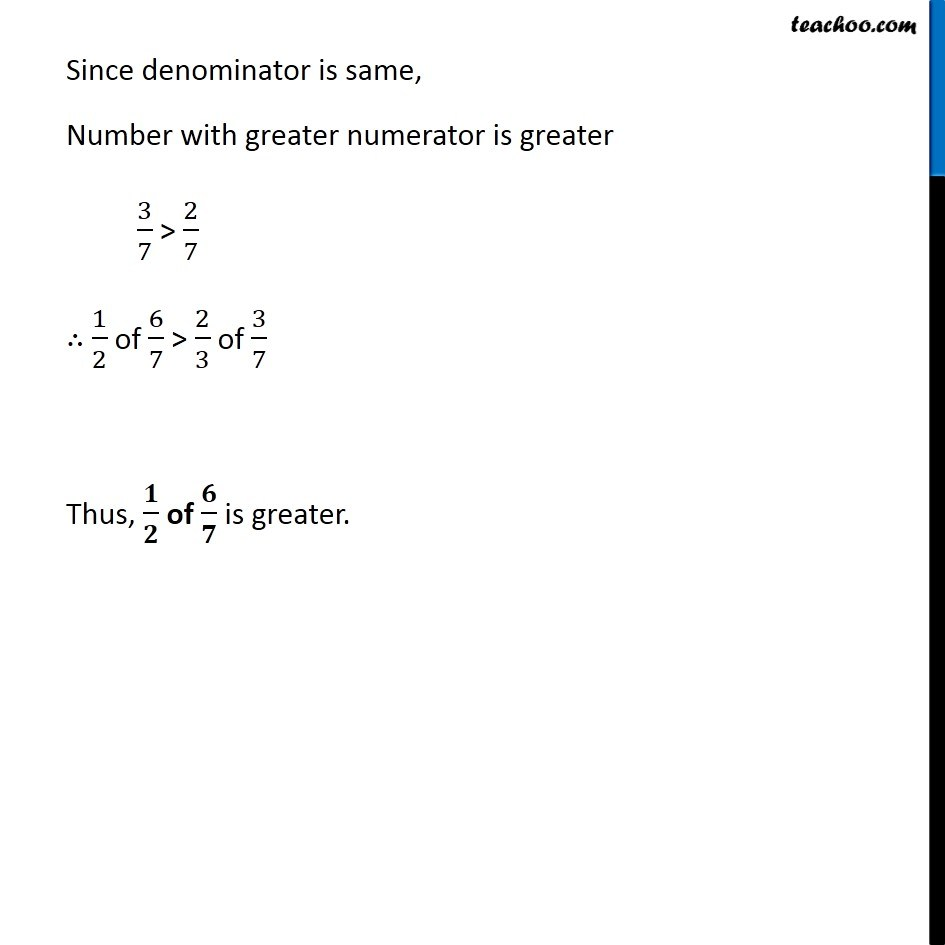Subscribe to our Youtube Channel - https://you.tube/teachoo

1. Chapter 2 Class 7 Fractions and Decimals
2. Concept wise
3. Multiplication of Fractions

Transcript

Ex 2.3, 4 Which is greater: (i) 2/7 of 3/4 or 3/5 of 5/8 2/7 of 3/4 = 2/7 × 3/4 = (2 × 3)/(7 × 4) = 3/(7 × 12) = 𝟑/𝟏𝟒 3/5 of 5/8 = 3/5 × 5/8 = (3 × 5)/(5 × 8) = 𝟑/𝟖 Now, We have to find greater of 3/14 & 3/8 To compare fractions, we make denominator equal. Common denominator = LCM of 14 & 8 = 2 × 2 × 2 × 7 = 8 × 7 = 56 So, 3/14 = 3/8 = ∴ 21/56 > 12/56 i.e. 3/8 > 3/14 ∴ 3/5 of 5/8 > 2/7 of 3/7 So, 𝟑/𝟓 of 𝟓/𝟖 is greater. Ex 2.3, 4 Which is greater: (ii) 1/2 of 6/7 or 2/3 of 3/71/2 of 6/7 = 1/2 × 6/7 = (1 × 6)/(2 × 7) = 𝟑/𝟕 2/7 of 3/7 = 2/7 × 3/7 = (2 × 3)/(3 × 7) = 𝟐/𝟕 Now, We have to find greater of 3/7 & 2/7 Since denominator is same, Number with greater numerator is greater 3/7 > 2/7 ∴ 1/2 of 6/7 > 2/3 of 3/7 Thus, 𝟏/𝟐 of 𝟔/𝟕 is greater.

Multiplication of Fractions